# Going Round in Circles

Or : Multiplying by $$i$$.

The beginning of complex numbers is often attributed to Cardano (1501 – 1576).  Complex numbers provide a solution to the equation $$x^2 = -1$$.

They are an extension of the number system from $$\Bbb R$$ (the real numbers) to $$\Bbb C$$ (the complex numbers).

Complex numbers take the form :

$$z = a + bi$$,

where $$a,b$$ are real numbers and $$i = \sqrt -1$$.

The normal rules of arithmetic and algebra apply with the additional rule that :

$$i^2 = -1$$

When two complex numbers are multiplied together the real numbers, $$a$$ and $$b$$, act as scalars and the imaginary number, $$i$$, acts as a rotation about the origin, $$0$$, (counter-clockwise from the positive real axis). Multiplication by $$i$$ on its own leads to a rotation of $$\pi/2$$ radians in the complex plane.

\begin{align}1\cdot i & = i & = i\\ i\cdot i & = i^2 & = -1\\ -1\cdot i & = i^3 & = -i\\ -i\cdot i & = -i^2 & = 1 \end{align}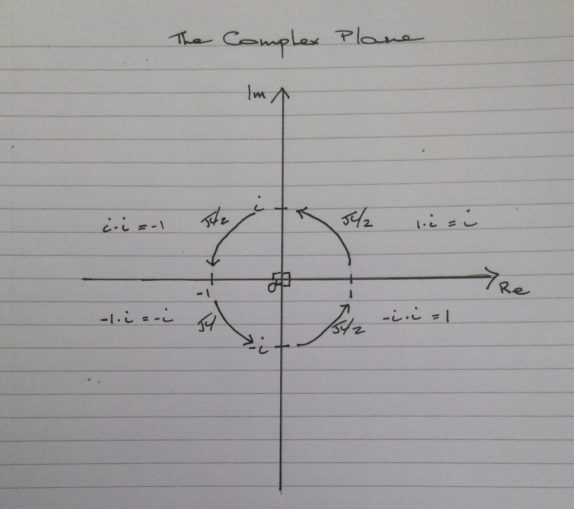Starting at any point on the real axis, (Re), and repeatedly mutilplying by $$i$$ traces larger or smaller circles.

The multiplication of two complex numbers gives another complex number.

Let :

$$z = a + bi$$ and $$w = c + di$$

Then :

\begin{align} zw & = (a + bi)(c + di)\\ & = ac + bdi^2 + adi + bci\\ & = ac-bd + (ad + bc)i \end{align}

Taking our starting point as one again, this time we multiply by $$1 + i$$ repeatedly.

\begin{align}1(1 + i) & = 1 + i\\ (1 + i)(1 + i) & = 2i\\ 2i(1 + i) & = -2 + 2i\\ (-2 + 2i)(1 + i) & = -4\\ -4(1 + i) & = -4-4i\\ (-4 – 4i)(1 + i) & = -8i\\ (-8i)(1 + i) & = 8-8i\\ (8 – 8i)(1 + i) & = 16 \end{align}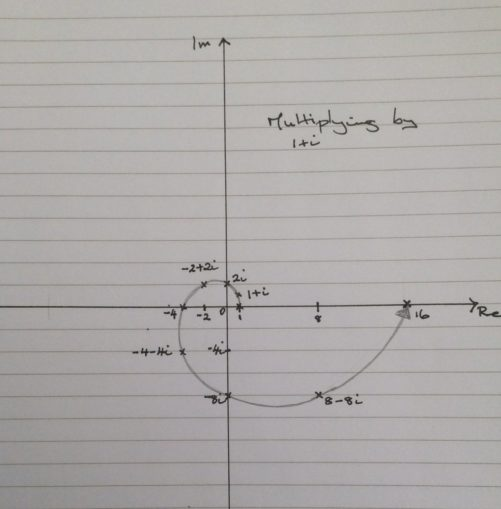We still have a rotational locus but now in the form of a spiral.

Each multiplication by $$1 + i$$ leads to a rotation by $$\pi/4$$ and an increase in distance from the origin by $$\sqrt 2$$.

This is because the point $$1 + i$$ is at a distance $$\sqrt 2$$ from the origin, $$0$$, (by Pythagorus) and the angle that this point makes to the positive real axis is arctan $$(1/1) = \pi/4$$, where arctan is the inverse of the tangent function.

We look at an example.

Let :

$$z = 3 + 4i$$ and $$w = 5 + 2i$$

Then :

$$zw = 7 + 26i$$,

by the above formula.

We can break it down to see what is happening.

$$(3 + 4i)5 = 15 + 20i$$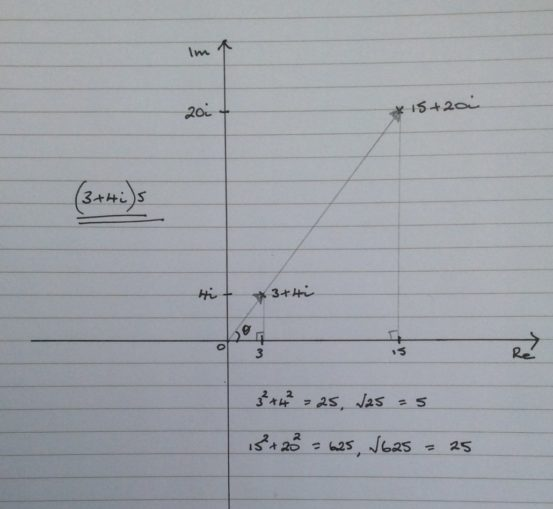The point has been scaled up by a factor of $$5$$ and the angle is left unchanged.

$$(3 + 4i)2i = -8 + 6i$$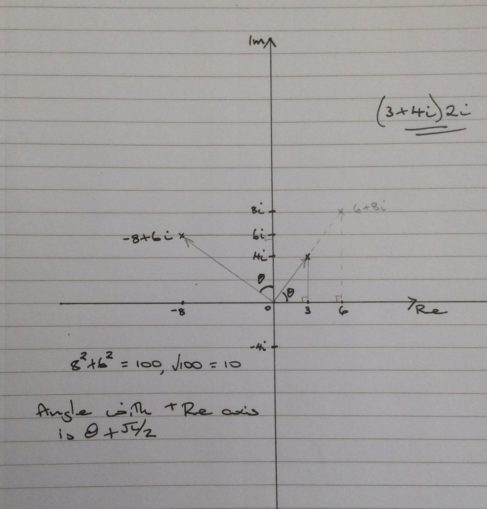The point has been scaled up by a factor of $$2$$ and rotated by an angle of $$\pi/2$$.

The addition of these two parts gives us the point $$7 + 26i$$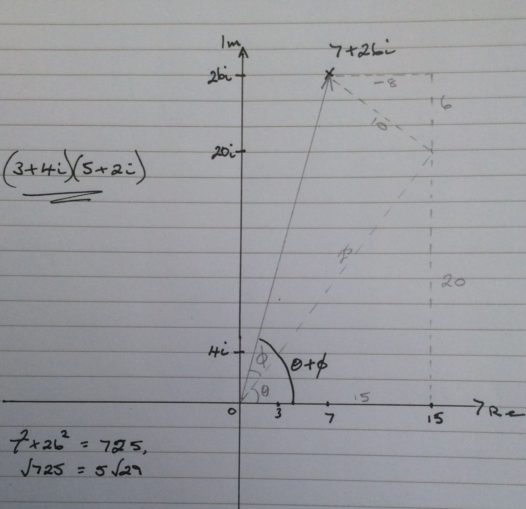In terms of distances and angles (the polar representation) :

$$z = (r_1, \theta), w = (r_2, \phi)$$ and $$zw = (r_1r_2, \theta + \phi)$$.

$$z = 3 + 4i = (5, \theta)$$, where $$\theta =$$arctan$$(4/3) \approx 0.9273$$ radians $$\approx 53.13^\circ$$

$$w = 5 + 2i = (\sqrt29, \phi)$$, where $$\phi =$$arctan$$(2/5) \approx 0.3805$$ radians $$\approx 21.80^\circ$$

$$zw = 7 +26i = (5\sqrt29, \theta + \phi)$$, where$$\theta + \phi =$$arctan$$(26/7) \approx 1.3078$$ radians $$\approx 74.93^\circ$$

Next time we will rotate in three different directions using the quaternions.

© OldTrout $$2019$$